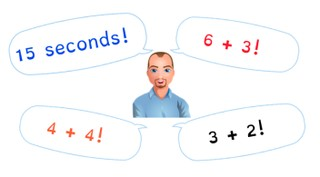Learn to quickly and correctly solve addition problems to 10.

No account needed.8,000 schools use Gynzy92,000 teachers use Gynzy1,600,000 students use Gynzy

## General

Students learn to solve addition problems to 10 quickly and correctly.

## Standards

CCSS.Math.Content.1.OA.C.6

## Learning objective

Students will be able to quickly and correctly solve addition problems up to 10 in their heads.

## Introduction

Practice three addition problems with the students by solving them using the visual support provided on the interactive whiteboard. For each problem, you can ask a student to come to the front of the class to drag the objects in the addition problem.

## Instruction

Explain to students what automaticity is, and what automaticity in addition problems means. Tell students that they are going to practice solving addition problems in their heads within a given time. The goal is to solve the addition problem within 5, 4, and even 3 seconds. Start by discussing the three problems, the first in 5 seconds, then 4, and finally in 3 seconds. Then ask students to solve the problems within those times and discuss the answers. Tell students that have trouble solving within the time, that this is something that improves with practice. Then challenge the class to solve the set of four problems within 20 seconds.

Check that students are able to quickly and correctly solve addition problems to 10 by asking the following questions:
- Why is it useful to be able to quickly solve addition problems?
- How can you make sure that you get better and faster at solving addition problems?

## Quiz

Students practice with sets of addition problems and are challenged to complete them in progressively less time.

## Closing

Explain the addition ball game: a student throws the ball to a classmate and says an addition problem. The student who catches the ball has to give the answer as quickly as possible. They then throw the ball to another classmate and name their own addition problem.

## Teaching tips

Students who have difficulty solving the addition problems within 5 seconds can first be given the task of practicing addition problems to 10 without any time limits. Then you can start by having them solve a problem in 10 seconds, then 8 seconds, etc.

## Instruction materials

A ball.

### The online teaching platform for interactive whiteboards and displays in schools

• Save time building lessons

• Manage the classroom more efficiently

• Increase student engagement